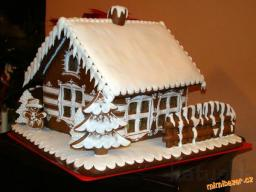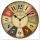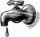Janka and Marienka calculated that there are 210 gingerbreads on the gingerbread house. Janko ate one-seventh of all gingerbreads, and Marienka ate a third less than Janko. How many gingerbreads remained in the gingerbread house?

Result

n =  160

#### Solution:

$J = 210/7 = 30 \ \\ M = (1-1/3) \cdot \ J = (1-1/3) \cdot \ 30 = 20 \ \\ \ \\ n = 210-J-M = 210-30-20 = 160$Our examples were largely sent or created by pupils and students themselves. Therefore, we would be pleased if you could send us any errors you found, spelling mistakes, or rephasing the example. Thank you!

Leave us a comment of this math problem and its solution (i.e. if it is still somewhat unclear...):Be the first to comment!#### Following knowledge from mathematics are needed to solve this word math problem:

Need help calculate sum, simplify or multiply fractions? Try our fraction calculator.

## Next similar math problems:

1. CakesOn the bowl were a few cakes. Jane ate one-third of them, Dana ate a quarter of those cakes that remained. a) What part (of the original number of cakes) Dana ate? b) At least how many cakes could be (initially) on thebowl?
2. Flood waterFlood waters in some US village meant that the homes had to evacuate 364 people. 50 of them stayed at elementary schools, 59 them slept with their friends and others went to relatives. How many people have gone to relatives?
3. SchoolThere are 150 pupils in grade 5 . 2/3 of it are female. By what fractions are the males?
4. Wood 11Father has 12 1/5 meters long wood. Then I cut the wood into two pieces. One part is 7 3/5 meters long. Calculate the length of the other wood?
5. In fractionsAn ant climbs 2/5 of the pole on the first hour and climbs 1/4 of the pole on the next hour. What part of the pole does the ant climb in two hours?
6. Lengths of the poolMiguel swam 6 lengths of the pool. Mat swam 3 times as far as Miguel. Lionel swam 1/3 as far as Miguel. How many lengths did mat swim?
7. Fraction and a decimalWrite as a fraction and a decimal. One and two plus three and five hundredths
8. ZdeněkZdeněk picked up 15 l of water from a 100-liter full-water barrel. Write a fraction of what part of Zdeněk's water he picked.Added together and write as decimal number: LXVII + MLXIV
10. Mixed2improperWrite the mixed number as an improper fraction. 166 2/3
11. Fraction to decimalWrite the fraction 3/22 as a decimal.
12. Pounds3 pounds subtract 1/3 of a pound.
13. MinutesDetermine the difference in minutes: T1 = 2 3/20 h T2 = 2.3 hAdd this two mixed numbers: 1 5/6 + 2 2/11=At the florist are 50 tulips and 5 times less roses. How many flowers are in flower shop?Mom bake cookies. Rolo took 2/9 of all cookies, Michal 3/9. How many cookies ate Rolo if Michal had 9.For one day flows 148 l of water out of the tap. How much water will flow out for 3/4 day?